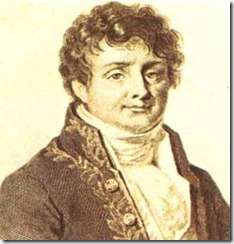## Motivation and Background:

In mathematics a transform is an operator applied to a function so that under the transform certain operations are simplified. In higher mathematics this idea is applied to functions in order to solve certain types of differential equations, for example, the Laplace transform.Another type of transform used to simply the process of solving differential equations is called the Fourier transform. Jean Baptiste Joseph Fourier (March 21, 1768 - May 16, 1830) was a French mathematician and physicist who is best known for initiating the investigation of Fourier series and their application to problems of heat flow. The Fourier transform is also named in his honor.

Fourier analysis has many scientific applications — in physics, number theory, combinatorics, signal processing, imaging, probability theory, statistics, option pricing, cryptography, numerical analysis, acoustics, oceanography, optics and diffraction, geometry, and other areas. When processing signals, such as audio, radio waves, light waves, seismic waves, and even images, Fourier analysis can isolate individual components of a compound waveform, concentrating them for easier detection and/or removal. For example, Telephone dialing; the touch-tone signals for each telephone key, when pressed, are each a sum of two separate tones (frequencies). Fourier analysis can be used to separate (or analyze) the telephone signal, to reveal the two component tones and therefore which button was pressed.

## Contents:

INTEGRAL TRANSFORM

FOURIER INTEGRAL THEOREM

FOURIER SINE AND COSINE INTEGRALS

FOURIER TRANSFORMS COMPLEX FOURIER TRANSFORMS

INVERSION FORMULA FOR THE COMPLEX FOURIER TRANSFORM

FOURIER SINE TRANSFORMS

FOURIER COSINE TRANSFORMS

PROPERTIES

• Linearity Property
• Change of Scale Property
• Shifting Property ( Shifting in x )
• Shifting in respect of s
• Modulation Theorem
• Conjugate Symmetry Property
• Transform of Derivatives
• Derivatives of the Transform
• Convolution Theorem
• Parseval’s Identity (or) Energy Theorem

FINITE FOURIER TRANSFORMS

Problems

Question Bank

1.